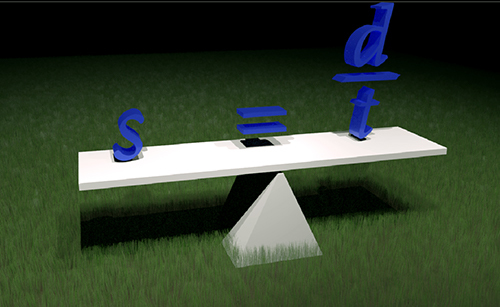• ### Browse All Lessons

##### Assign Lesson

Help Teaching subscribers can assign lessons to their students to review online!

 Tweet# Solving for VariablesAlgebra is all about finding missing information. Information which is unknown or can change is represented by variables. Typically letters of the alphabet are used to symbolise variables.

You can think of a variable as a mail box, each one having a letter on it. Generic equations can be written and then used repeatedly by putting different numbers into the “mail boxes.” For instance, speed is calculated by dividing the distance traveled by the time taken to travel. An equation to represent this could be $s=d/t$. If we drive $200$ km in $2$ hr we can put the numbers into the appropriate variables $d=200km$ and $t=2hr$ to get $s=(200km)/(2hr)$ which means that the average speed was $100$ km/hr. We can use the same equation if we travel $320$ miles in $5$ hr which would then become $s=(320mi)/(5hr)$ meaning that the average speed would be $64$ mph.

The power of using variables is that they are efficient, we can use one equation to represent many different scenarios. The term variable just means that what the letter represents can vary or change.

One very important thing to remember is that within one equation a variable will always have the same value. In other words, any time we use an $x$ it must be the same value as any other $x$ in the equation.

Although you have probably been solving simple equations using variables for a while, the equations have most likely been given in a form that is created to solve for the variable you want. In the scenario above finding speed, what if we knew the speed and time but wanted to find the distance? The equation above, $s=d/t$, is solving for $s$ but now we need to solve for $d$. This lesson is focused on rearranging equations so that we can solve for specific variables. The steps for solving for $d$ would be…
$s=d/t$ Begin by stating the original equation
$st=dt/t$ Since $d$ is attached to $t$ by division we must multiply both sides by $t$
$st=d$ Since $t/t=1$ they cancel
$d=st$ Always begin with the variable we are solving for and put the rest of the variables alphabetically

It is easy to check if the equation still works. Let's use the original speed and time to see if the distance is correct. Plug the numbers back in to get $d=100 xx2$ and yes, the distance is $200$ km.

Basic Rules
•What ever you do to one side of the equation you must do to both sides!
•When one term is attached to another one and you want to move it, you must use the opposite operation.
–If it is added you must subtract - If it is subtracted you must add
–If it is multiplied you must divide – If it is divided you must multiply
–If it is squared you must square root – If it is square rooted you must square

Directions for this Lesson:
Watch the video below, complete the practice questions, and then complete the worksheet.

Required Video:

Related Worksheets: